Test: Poly Phase AC Circuit- 2

# Test: Poly Phase AC Circuit- 2

Test Description

## 15 Questions MCQ Test Topicwise Question Bank for Electrical Engineering | Test: Poly Phase AC Circuit- 2

Test: Poly Phase AC Circuit- 2 for Electrical Engineering (EE) 2023 is part of Topicwise Question Bank for Electrical Engineering preparation. The Test: Poly Phase AC Circuit- 2 questions and answers have been prepared according to the Electrical Engineering (EE) exam syllabus.The Test: Poly Phase AC Circuit- 2 MCQs are made for Electrical Engineering (EE) 2023 Exam. Find important definitions, questions, notes, meanings, examples, exercises, MCQs and online tests for Test: Poly Phase AC Circuit- 2 below.
Solutions of Test: Poly Phase AC Circuit- 2 questions in English are available as part of our Topicwise Question Bank for Electrical Engineering for Electrical Engineering (EE) & Test: Poly Phase AC Circuit- 2 solutions in Hindi for Topicwise Question Bank for Electrical Engineering course. Download more important topics, notes, lectures and mock test series for Electrical Engineering (EE) Exam by signing up for free. Attempt Test: Poly Phase AC Circuit- 2 | 15 questions in 30 minutes | Mock test for Electrical Engineering (EE) preparation | Free important questions MCQ to study Topicwise Question Bank for Electrical Engineering for Electrical Engineering (EE) Exam | Download free PDF with solutions
 1 Crore+ students have signed up on EduRev. Have you?
Test: Poly Phase AC Circuit- 2 - Question 1

### Three equal resistances connected in star take a line current of 10 A when fed from 400 V, 50 Hz source. If the load resistances are reconnected in delta, the line current would be

Detailed Solution for Test: Poly Phase AC Circuit- 2 - Question 1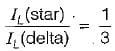or,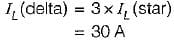Test: Poly Phase AC Circuit- 2 - Question 2

### The minimum number of wattmeters required to measure the real power in an n-phase system with unbalanced load is

Test: Poly Phase AC Circuit- 2 - Question 3

### The input to the 3-phase, 50 Hz circuit shown in figure below is 100 V. For a phase sequence of ABC, the wattmeters would read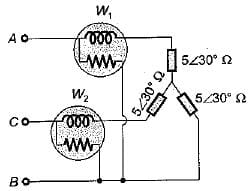Detailed Solution for Test: Poly Phase AC Circuit- 2 - Question 3

W1 = VLIL cos (30 + φ)
and W2 = \/LIL cos (30 - φ)
Here,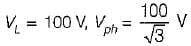and φ = 30°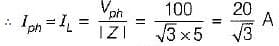Now,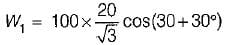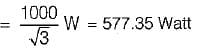and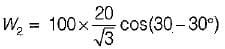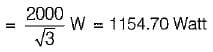Test: Poly Phase AC Circuit- 2 - Question 4

Three-phase power can be measured by two-wattmeter method in case of

Test: Poly Phase AC Circuit- 2 - Question 5

In the case of power measured by two-wattmeter method in a balanced 3-phase system with a pure capacitive load

Detailed Solution for Test: Poly Phase AC Circuit- 2 - Question 5

W1 = VIL cos (30° + φ)
and W= VIL cos(30° - φ)
When the load is purely capacitance, then φ = - 90°.
So, W1 = VLI cos(30°- 90°)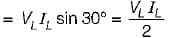and W2 = VLIL cos (30° + 90°)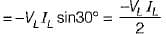Hence, |W1| = |W2| but W1 = - W2

Test: Poly Phase AC Circuit- 2 - Question 6

Measurement of power factor of a 3-phase system by two-wattmeter method can be obtained in case of

Test: Poly Phase AC Circuit- 2 - Question 7

Two wattmeters used to measure power of a 3-phase balanced load reads W1, and W2. The reactive Dower drawn bv the load is

Detailed Solution for Test: Poly Phase AC Circuit- 2 - Question 7

We know that:
(W- W2) = VLILsin φ = Q1 - φ
So, Q3 - φ = √3(W1 - W2)

Test: Poly Phase AC Circuit- 2 - Question 8

Two wattmeters used to measure power of a 3-phase balanced load reads W1 and W2. The' reactive Dower drawn bv the load is

Detailed Solution for Test: Poly Phase AC Circuit- 2 - Question 8

We know that:
(W1 - W2) = VL IL sin φ = Q1-φ
so, Q3 - φ = √3(W1 - W2)

Test: Poly Phase AC Circuit- 2 - Question 9

A three-phase 220 V supply is applied to a balanced Δ-connected three phase load. The phase current being Iab =. 10∠-30°A as shown in the given figure, The total power received by the Δ-load is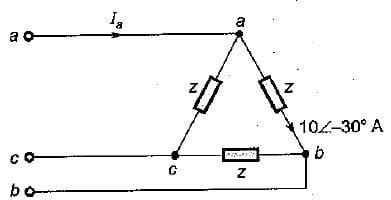Detailed Solution for Test: Poly Phase AC Circuit- 2 - Question 9

Iab = 10∠-30°A
So, Ibc = 10∠-150°A
and Ica = 10∠-270° A
Phase current,
Ia = Iab - Ica = (10∠-30° - 10∠-270°) A
= 10[cos 30° - jsin 30° - cos 270° + jsin 270°]
= 10[0.866 - j0.5 - 0 - j1]
= 8.66 - j15 = 17.32 ∠-60° A
∴ Ia = 17.32 ∠-60°A
= √3 VLIL cos φL
= 3VPIP cos φph
= 3 x 220 x 10 x cos 30°
= 5715W

Test: Poly Phase AC Circuit- 2 - Question 10

The magnitude of line current in the circuit shown below is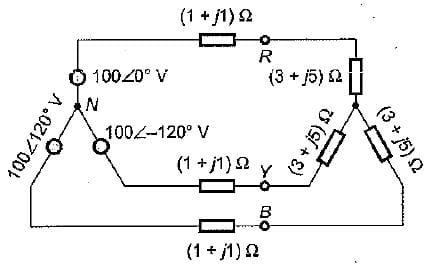Detailed Solution for Test: Poly Phase AC Circuit- 2 - Question 10

Impedance per phase
= 1 + j1 + 3 + j5
= (4 + j6) Ω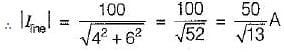Test: Poly Phase AC Circuit- 2 - Question 11

A three-phase, 400 V ac system is connected across a delta connected load having load resistance per phase of 200 Ω. If one of the phases of the delta connected load experiences an open circuit, then power consumed by the load will be

Detailed Solution for Test: Poly Phase AC Circuit- 2 - Question 11

Initially, in closed - Δ, power consumed by the load is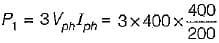= 2400W
Let, P2 be the power consumed in open delta.
then,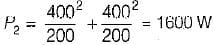Test: Poly Phase AC Circuit- 2 - Question 12

Assertion (A): Generated voltage or current wave have no odd harmonics.
Reason (R): The field system and armature coils of the generators are all symmetrical and so generates mostly symmetrical voltage wave.

Test: Poly Phase AC Circuit- 2 - Question 13

Assertion (A): RMS value of line voltage is more than √3 times of the rms value of phase voltage.
Reason (R): In a star-connected system, the line voltage contains no triple frequency harmonic content.

Test: Poly Phase AC Circuit- 2 - Question 14

Assertion (A): In a balanced 3-phase star connected system, line voltages are 30° ahead of the respective phase voltages.
Reason (R): The line voltages are √3 times of the respective phase voltages,

Test: Poly Phase AC Circuit- 2 - Question 15

Match the List - I (Power factor) with List - II (Wattmeter readings) for the measurement of power in a three-phase system using two-wattmeter method and select the correct answer using the codes given below the lists:
List-I
A. 0.5 lag
B. Unity
C. Zero
D. 0.866

List-II
1. W1 = W2
2. W1 = 2W2
3. W1 > 0,  W2 = 0
4. W1 > 0, W2 < 0

Codes: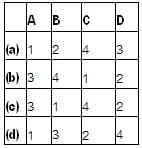Detailed Solution for Test: Poly Phase AC Circuit- 2 - Question 15

W1 = VLIL cos (30 + φ)
and W2 = VLIL cos (30 - φ)
By finding φ from cos φ, we can find the values of W1 and W2.

## Topicwise Question Bank for Electrical Engineering

207 tests
 Use Code STAYHOME200 and get INR 200 additional OFF Use Coupon Code
Information about Test: Poly Phase AC Circuit- 2 Page
In this test you can find the Exam questions for Test: Poly Phase AC Circuit- 2 solved & explained in the simplest way possible. Besides giving Questions and answers for Test: Poly Phase AC Circuit- 2, EduRev gives you an ample number of Online tests for practice

207 tests